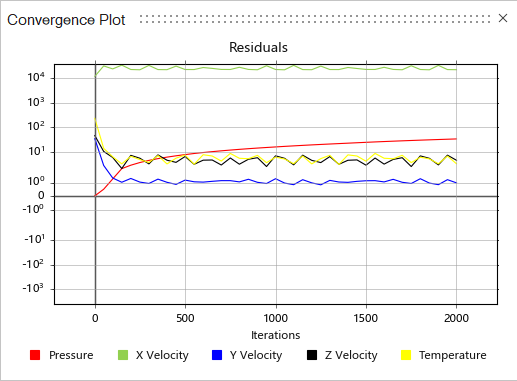# View Convergence Plots and Tables

Monitor the convergence of Engineering quantities and Equation residuals.

1. Run a Fluids simulation.
2. While the simulation is running, on the Fluids ribbon, select the View Convergence Plot tool in the Analyze Fluids tool group.The Convergence Plot window appears.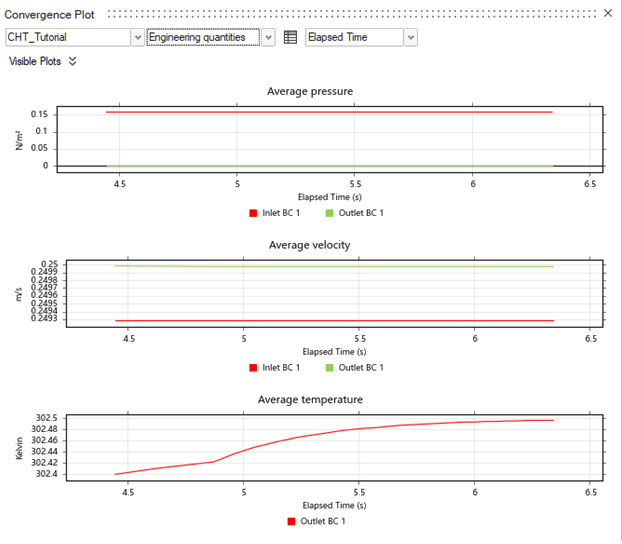3. Select to display Engineering quantities (default) or Numerical convergence results.
4. To view Engineering quantities options, select Engineering quantities from the dropdown menu.
To Do this
Select which convergence plots to view
• Select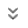next to Visible Plots, then enable or disable the following switches:
• Average pressure
• Average velocity
• Flow fraction
• Uniformity velocity
Display the convergence data in a table
• Click the Show Convergence Table icon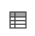. The data in the Convergence Plot window will display as a table in the modeling window.
Plot results over time (Seconds) Select Seconds from the dropdown menu.
Plot results over the Number of iterations Select Number of iterations from the dropdown menu.
5. To view Numerical convergence options, select Numerical convergence from the dropdown menu.
The equation residuals from the analysis appear.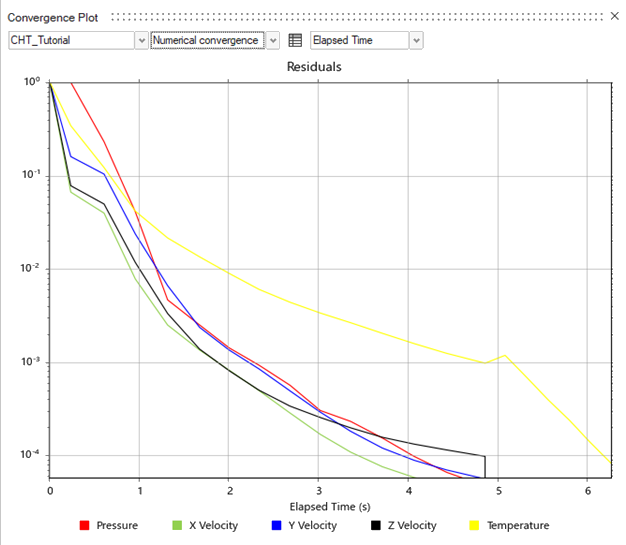To Do this
Plot results over time (Seconds) Select Seconds from the dropdown menu.
Plot results over the Number of iterations Select Number of iterations from the dropdown menu.
Tip:
• When the Numerical convergence curves drop below 1.e-4, you can stop the simulation in advance to review the results.
• If the Numerical convergence curves go up and down sharply, review and modify the model setup and run a new simulation to produce valid results.